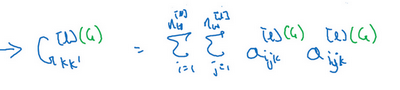## 第四周 特殊应用：人脸识别和神经风格转换（Special applications: Face recognition &Neural style transfer）

### 4.1 什么是人脸识别？（What is face recognition?）

（以下内容为演示视频内容）（林元庆语：我将尝试用Andrew的工卡骗过机器，看看发生什么，系统不会识别，系统拒绝识别。现在我要用我自己的脸，（系统语音：“欢迎您”）（林元庆顺利通过））

#视频结束### 4.2 One-Shot学习（One-shot learning）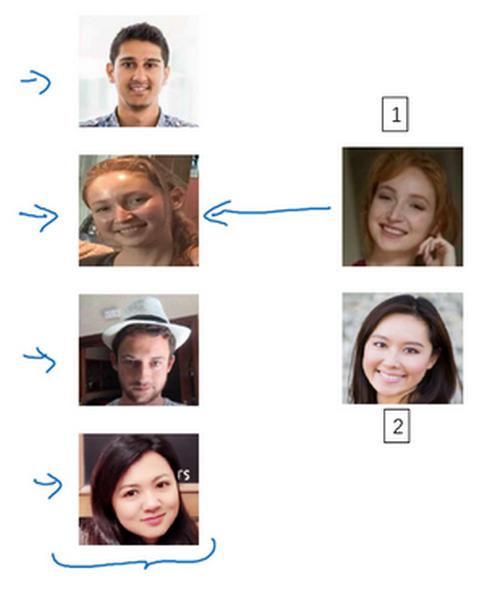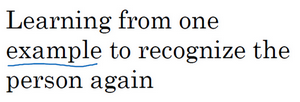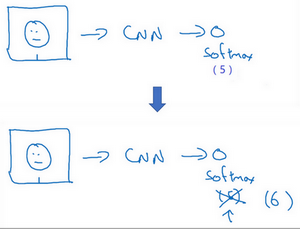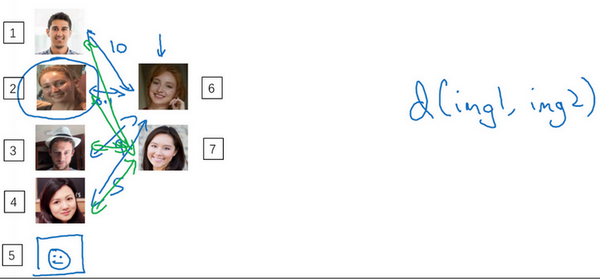### 4.3 Siamese 网络（Siamese network）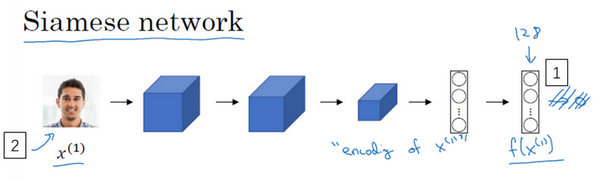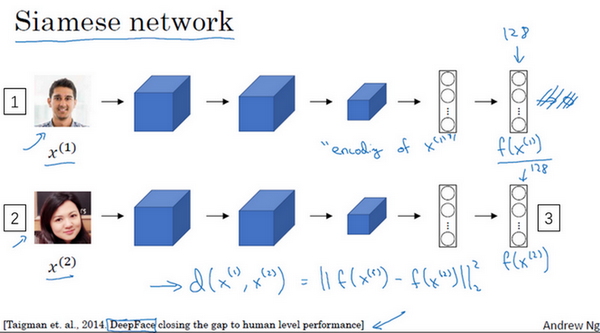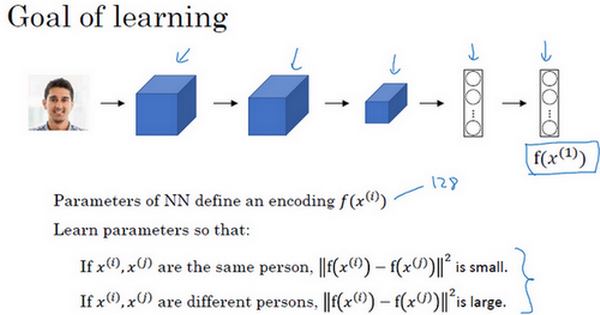### 4.4 Triplet 损失（Triplet 损失）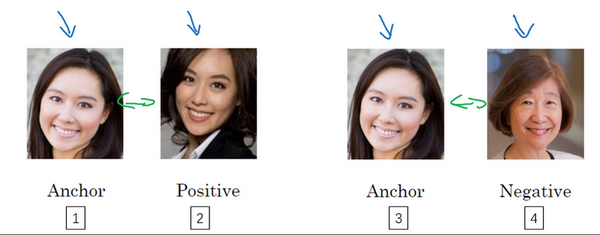$|| f(A) - f(P)||^{2} \leq ||f(A) - f(N)||^{2}$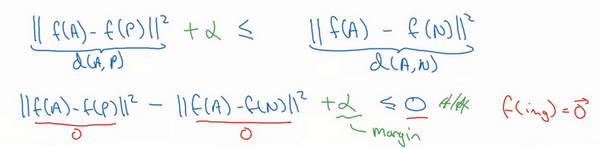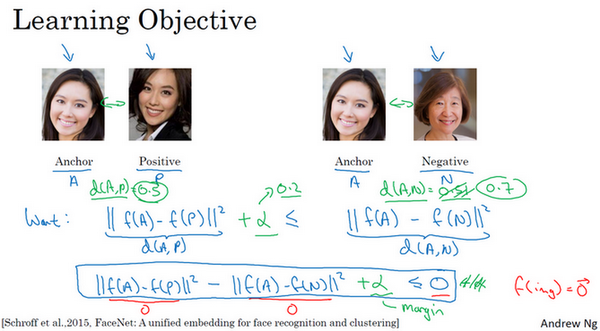$L( A,P,N) = max(|| f( A) - f( P)||^{2} -|| f( A) - f( N)||^{2} + a,0)$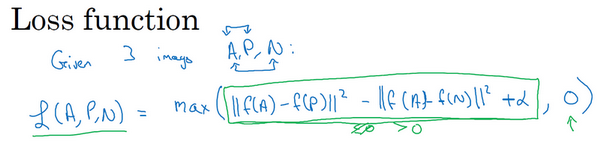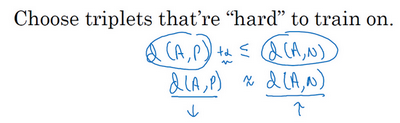Florian Schroff, Dmitry Kalenichenko, James Philbin (2015). FaceNet: A Unified Embedding forFace Recognition and Clustering### 4.5 人脸验证与二分类（Face verification and binary classification）

Triplet loss是一个学习人脸识别卷积网络参数的好方法，还有其他学习参数的方法，让我们看看如何将人脸识别当成一个二分类问题。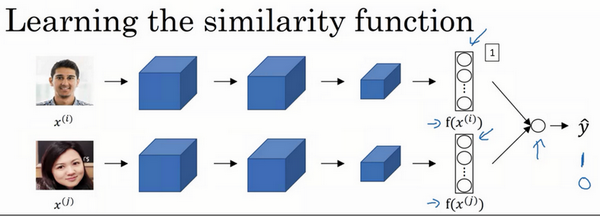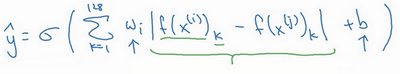$\hat y = \sigma(\sum_{k = 1}^{128}{w_{i}| f( x^{( i)})_{k} - f( x^{( j)})_{k}| + b})$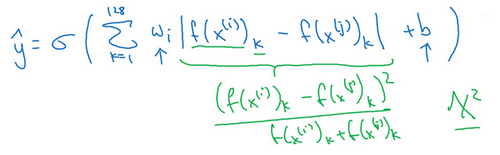Yaniv Taigman, Ming Yang, Marc'Aurelio Ranzato, Lior Wolf (2014). DeepFace:Closing the gap to human-level performance in face verification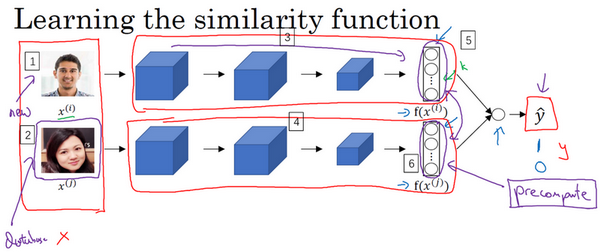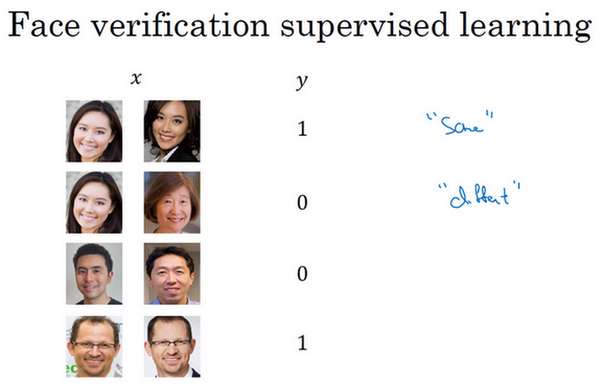### 4.6 什么是神经风格迁移？（What is neural style transfer?）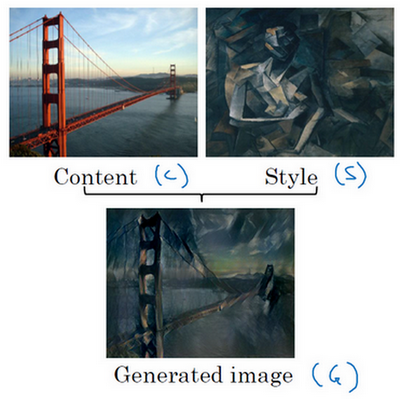### 4.7 CNN特征可视化（What are deep ConvNets learning?）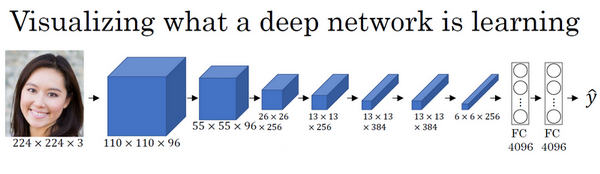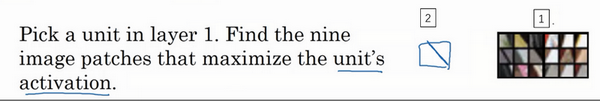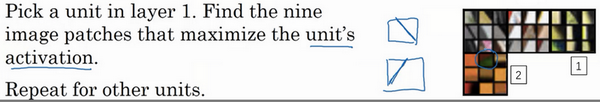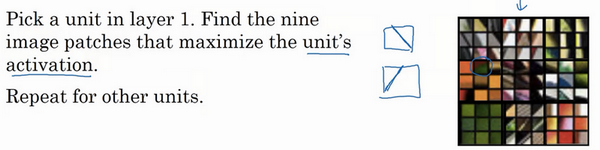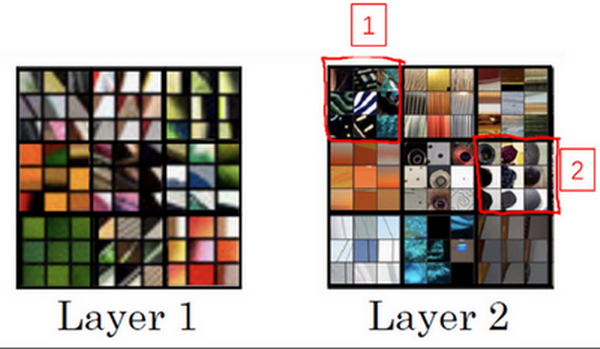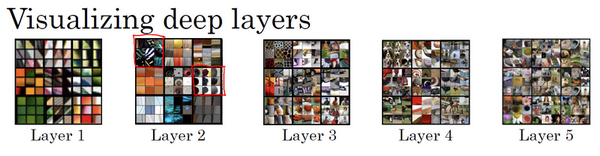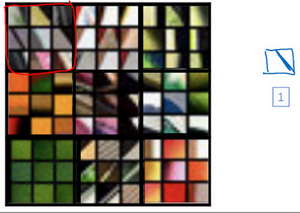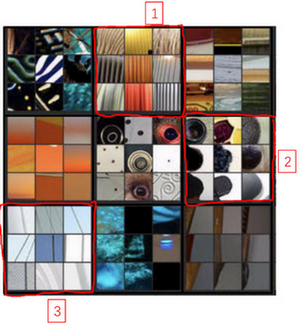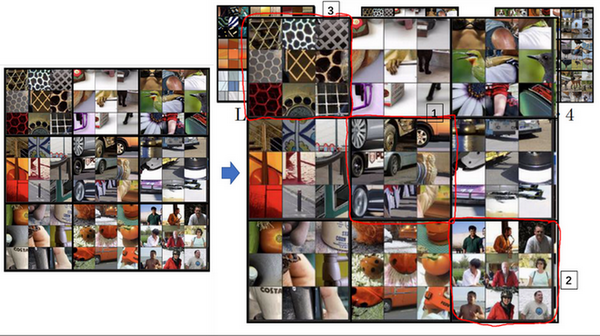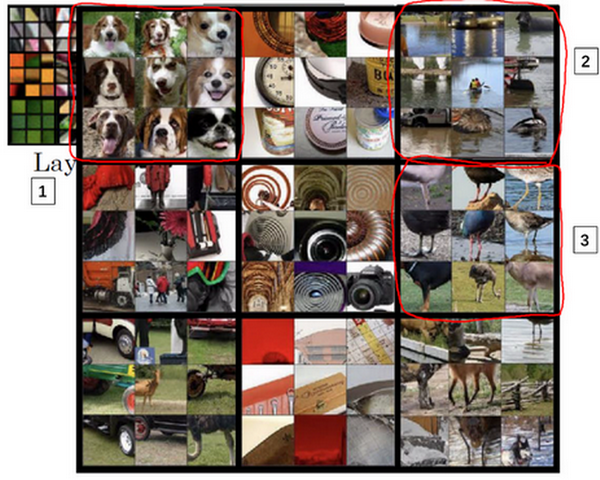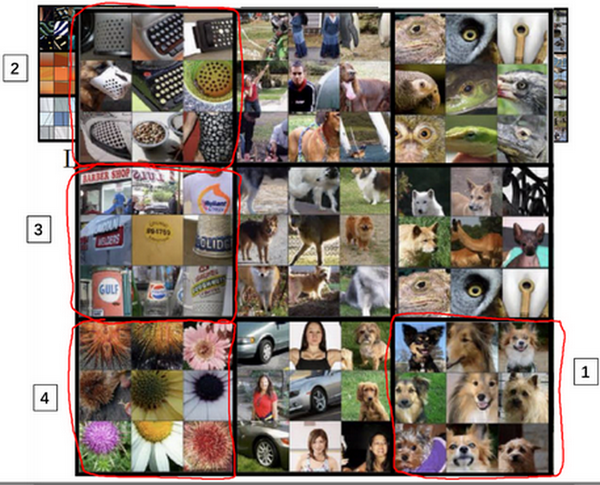### 4.8 代价函数（Cost function）$J_{\text{content}}(C,G)$

$J_{\text{style}}(S,G)$

$J( G) = a J_{\text{content}}( C,G) + \beta J_{\text{style}}(S,G)$

Leon A. Gatys, Alexander S. Ecker, Matthias Bethge, (2015). A Neural Algorithm of Artistic Style (https://arxiv.org/abs/1508.06576)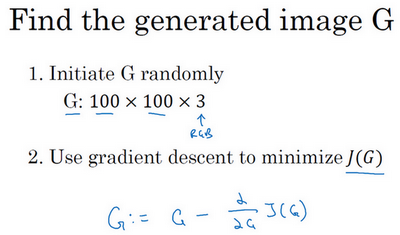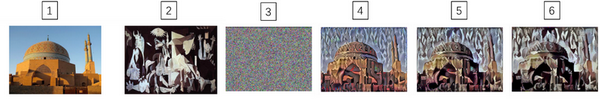### 4.9 内容代价函数（Content cost function）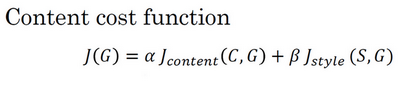$J( G) = \alpha J_{\text{content}}( C,G) + \beta J_{\text{style}}(S,G)$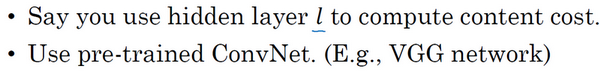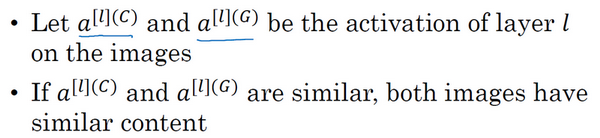$J_{\text{content}}( C,G) = \frac{1}{2}|| a^{[l][C]} - a^{[l][G]}||^{2}$

### 4.10 风格代价函数（Style cost function）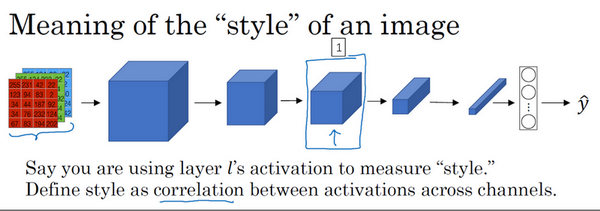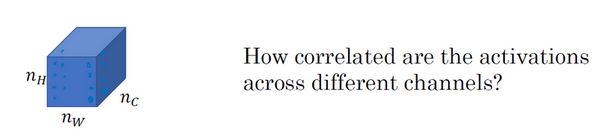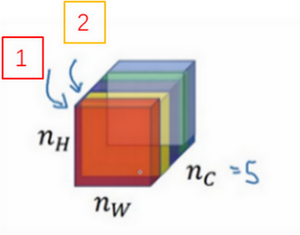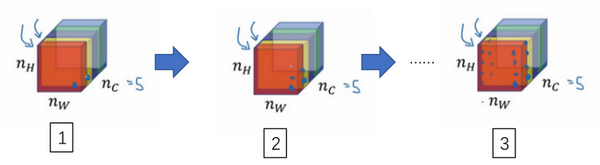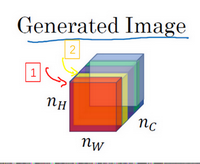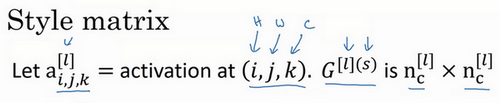$G_{kk^{'}}^{[l]( S)} = \sum_{i = 1}^{n_{H}^{[l]}}{\sum_{j = 1}^{n_{W}^{[l]}}{a_{i,\ j,\ k}^{[l](S)}a_{i,\ j,\ k^{'}}^{[l](S)}}}$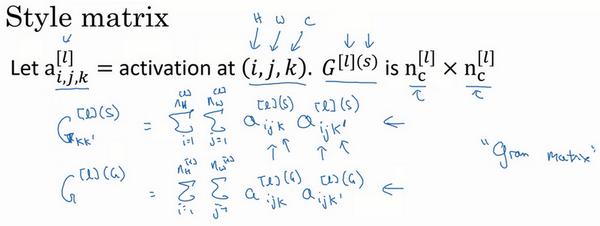$G_{kk^{'}}^{[l]( G)} = \sum_{i = 1}^{n_{H}^{[l]}}{\sum_{j = 1}^{n_{W}^{[l]}}{a_{i,\ j,\ k}^{[l](G)}a_{i,\ j,\ k^{'}}^{[l](G)}}}$

$a_{i,\ j,\ k}^{[l](S)}$$a_{i, j,k}^{[l](G)}$中的上标$(S)$$(G)$分别表示在风格图像$S$中的激活项和在生成图像$G$的激活项。我们之所以用大写字母$G$来代表这些风格矩阵，是因为在线性代数中这种矩阵有时也叫Gram矩阵，但在这里我只把它们叫做风格矩阵。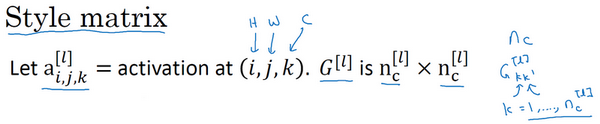$G_{kk^{'}}^{[l]} = \sum_{i = 1}^{n_{H}^{[l]}}{\sum_{j = 1}^{n_{W}^{[l]}}{a_{i,\ j,\ k}^{[l]}a_{i,\ j,\ k^{'}}^{[l]}}}$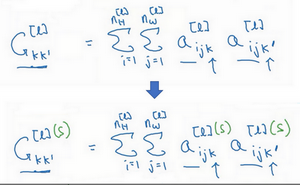$G_{kk^{'}}^{[l]( S)} = \sum_{i = 1}^{n_{H}^{[l]}}{\sum_{j = 1}^{n_{W}^{[l]}}{a_{i,\ j,\ k}^{[l](S)}a_{i,\ j,\ k^{'}}^{[l](S)}}}$

$G_{kk^{'}}^{[l]( G)} = \sum_{i = 1}^{n_{H}^{[l]}}{\sum_{j = 1}^{n_{W}^{[l]}}{a_{i,\ j,\ k}^{[l](G)}a_{i,\ j,\ k^{'}}^{[l](G)}}}$